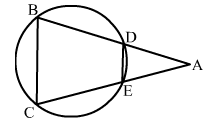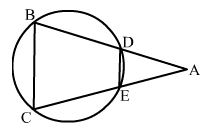# In the given figure ∆ABC is an isosceles triangle in which AB = AC and a circle passing through B and C intersects AB and AC at D and E respectively.

Question:

In the given figure ABC is an isosceles triangle in which AB = AC and a circle passing through B and C intersects AB and AC at D and E respectively. Prove that DE || BC.Solution:ABC is an isosceles triangle.
Here, AB = AC
∴ ∠ACB = ∠ABC   ...(i)